• 深度循环神经网络和双向循环神经网络 1、深度循环神经网络 本章到目前为止介绍的循环神经网络只有一个单向的隐藏层，在深度学习应用里，我们通常会用到含有多个隐藏层的循环神经网络，也称作深度循环神经网络。图...
深度循环神经网络和双向循环神经网络
1、深度循环神经网络
本章到目前为止介绍的循环神经网络只有一个单向的隐藏层，在深度学习应用里，我们通常会用到含有多个隐藏层的循环神经网络，也称作深度循环神经网络。图6.11演示了一个有 个隐藏层的深度循环神经网络，每个隐藏状态不不断传递至当前层的下一时间步和当前时间步的下一层。
如下图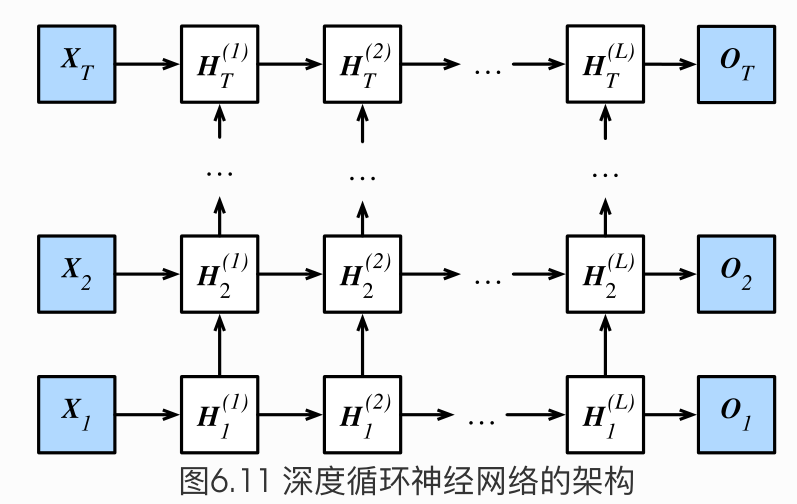$\boldsymbol{H}_t^{(1)} = \phi(\boldsymbol{X}_t \boldsymbol{W}_{xh}^{(1)} + \boldsymbol{H}_{t-1}^{(1)} \boldsymbol{W}_{hh}^{(1)} + \boldsymbol{b}_h^{(1)})\\\boldsymbol{H}_t^{(\ell)} = \phi(\boldsymbol{H}_t^{(\ell-1)} \boldsymbol{W}_{xh}^{(\ell)} + \boldsymbol{H}_{t-1}^{(\ell)} \boldsymbol{W}_{hh}^{(\ell)} + \boldsymbol{b}_h^{(\ell)})\\\boldsymbol{O}_t = \boldsymbol{H}_t^{(L)} \boldsymbol{W}_{hq} + \boldsymbol{b}_q$
我们之前学的到的循环神经网络都单隐藏层的，如下图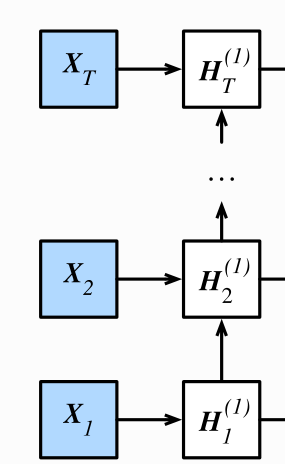具体来说就是，将X1输入到第一个循环神经元H1，这个rnn神经元可以是GRU也可以是LSTM，这里的H1是第一个时间步输出的隐藏状态，其将作为第二个时间步的输入隐藏状态，隐藏状态就这样一直往上传，直到HT。每个Hi（i=1,2,3…T）之后都会接一个输出层output（Oi  i=1,2,3…T)，这就是之前的单隐层RNN。

对于深度循环神经网络，只是将第一层的每个隐藏状态Hi（i=1,2,3…T），做为第二层的输入，最后。H的上标表示层数，下标表示时间步数。在第L层之后做输出。

部分代码 加上num_layers即可食用 (两层的)
num_hiddens=256
num_epochs, num_steps, batch_size, lr, clipping_theta = 160, 35, 32, 1e2, 1e-2
pred_period, pred_len, prefixes = 40, 50, ['分开', '不分开']

#lr = 1e-2  注意调整学习率
#只需要在nn.LSTM原来的基础上加上参数num_layers=2,即可变成两层的循环神经网络(输入层+两个隐层+输出层)
gru_layer = nn.LSTM(input_size=vocab_size, hidden_size=num_hiddens,num_layers=2)
model = d2l.RNNModel(gru_layer, vocab_size).to(device)
d2l.train_and_predict_rnn_pytorch(model, num_hiddens, vocab_size, device,
corpus_indices, idx_to_char, char_to_idx,
num_epochs, num_steps, lr, clipping_theta,
batch_size, pred_period, pred_len, prefixes)

部分代码  (六层的)
gru_layer = nn.LSTM(input_size=vocab_size, hidden_size=num_hiddens,num_layers=6)
model = d2l.RNNModel(gru_layer, vocab_size).to(device)
d2l.train_and_predict_rnn_pytorch(model, num_hiddens, vocab_size, device,
corpus_indices, idx_to_char, char_to_idx,
num_epochs, num_steps, lr, clipping_theta,
batch_size, pred_period, pred_len, prefixes)

实践证明，上边num_layers=2的结果要比num_layers=6的结果要好很多的，所以说深度循环网络不是越深越好。
越深表示模型越复杂，对数据集的要求更高，内容更加抽象。所以参数的设置还要看实践和经验。
小结

在深度循环神经网络中，隐藏状态的信息不断传递至当前层的下一时间步和当前时间步的下一层。

2、双向循环神经网络
之前介绍的循环神经网络模型都是假设当前时间步是由前面的较早时间步的序列决定的，因此它们都将信息通过隐藏状态从前往后传递。有时候，当前时间步也可能由后⾯面时间步决定。例如，当我们写下一个句子时，可能会根据句子后面的词来修改句子前面的用词。双向循环神经网络通过增加从后往前传递信息的隐藏层来更灵活地处理这类信息。
图6.12演示了一个含单隐藏层的双向循环神经网络的架构。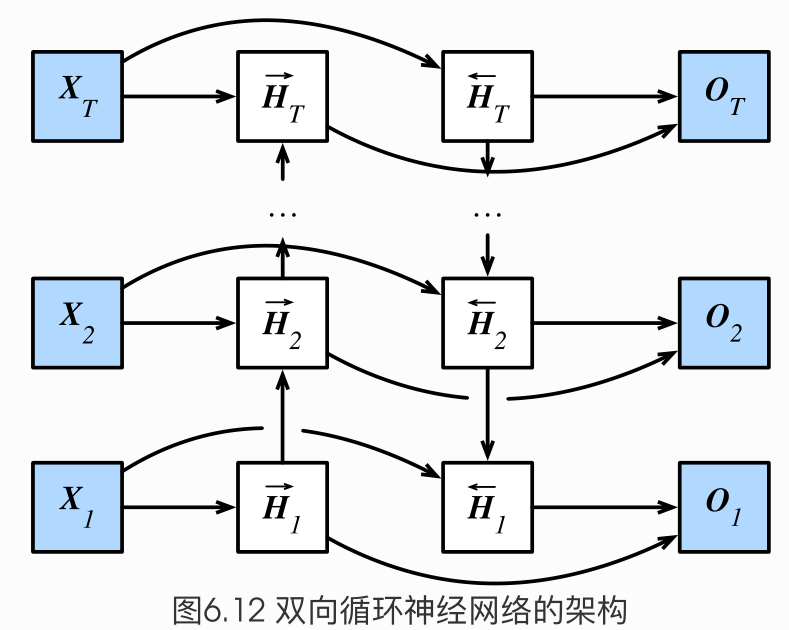\begin{aligned} \overrightarrow{\boldsymbol{H}}_t &= \phi(\boldsymbol{X}_t \boldsymbol{W}_{xh}^{(f)} + \overrightarrow{\boldsymbol{H}}_{t-1} \boldsymbol{W}_{hh}^{(f)} + \boldsymbol{b}_h^{(f)})\\\overleftarrow{\boldsymbol{H}}_t &= \phi(\boldsymbol{X}_t \boldsymbol{W}_{xh}^{(b)} + \overleftarrow{\boldsymbol{H}}_{t+1} \boldsymbol{W}_{hh}^{(b)} + \boldsymbol{b}_h^{(b)}) \end{aligned}
$\boldsymbol{H}_t=(\overrightarrow{\boldsymbol{H}}_{t}, \overleftarrow{\boldsymbol{H}}_t)$
$\boldsymbol{O}_t = \boldsymbol{H}_t \boldsymbol{W}_{hq} + \boldsymbol{b}_q$

我们可以分别计算正向隐藏状态（→）和反向隐藏状态（←）

两个方向的隐藏层的连接方式是 concat 拼接起来，蕴含了两个方向的信息 ，根据上图可以想成一个信息往上传，一个信息往下传。

不同方向上的隐藏单元个数也可以不同。

双向循环神经网络的好处：比如 X 1 到 X T 是一句话，本来我们只考虑了一个方向，即前面的字对后面的字影响。如果是双向的，那么每个字都会受到前面的字和后面的字的影响

很简单 加上 bidirectional=True 即可食用：
num_hiddens=128
num_epochs, num_steps, batch_size, lr, clipping_theta = 160, 35, 32, 1e-2, 1e-2
pred_period, pred_len, prefixes = 40, 50, ['分开', '不分开']

lr = 1e-2 # 注意调整学习率
#设置bidirectional=True 即可构建双向循环神经网络
gru_layer = nn.GRU(input_size=vocab_size, hidden_size=num_hiddens,bidirectional=True)
model = d2l.RNNModel(gru_layer, vocab_size).to(device)
d2l.train_and_predict_rnn_pytorch(model, num_hiddens, vocab_size, device,
corpus_indices, idx_to_char, char_to_idx,
num_epochs, num_steps, lr, clipping_theta,
batch_size, pred_period, pred_len, prefixes)

实践证明，双向带来了更多得参数，效果未必会好。
双向RNN是很常见的，但是否适用还需要实践来看效果。
小结
双向循环神经网络在每个时间步的隐藏状态同时取决于该时间步之前和之后的子序列（包括当前
时间步的输入）。


展开全文• 文章目录门控循环神经网络GRU长短期记忆LSTM深度循环神经网络双向循环神经网络 门控循环神经网络GRU 该网络的提出也同样是为了应对普通RNN的梯度问题 基本原理看这一篇就能懂：转载自知乎 简单来说，GRU就是通过一个...
文章目录门控循环神经网络GRU长短期记忆LSTM深度循环神经网络双向循环神经网络
门控循环神经网络GRU
该网络的提出也同样是为了应对普通RNN的梯度问题
基本原理看这一篇就能懂：转载自知乎
简单来说，GRU就是通过一个重置门控和一个更新门控来实现对原隐藏信息的选择性遗忘和对当前节点信息的选择性记忆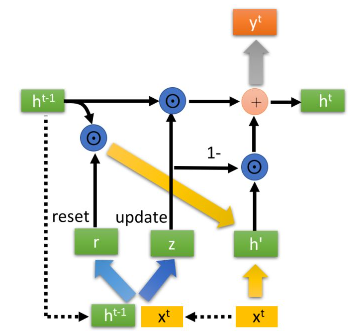可以看到其中的r和z分别是重置门控和更新门控，首先由上一层的隐藏信息ht-1和当先层的输入分别得到门控r和z，激活函数选择sigmoid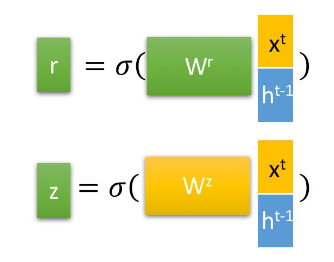接着使用重置门控r来重置上一层的隐藏信息ht-1得到ht-1’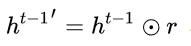这个运算符号是指Hadamard Product，也就是操作矩阵中对应的元素相乘，因此要求两个相乘矩阵是同型的。然后将更新后的ht-1’与输入X拼接，运用tanh得到h’。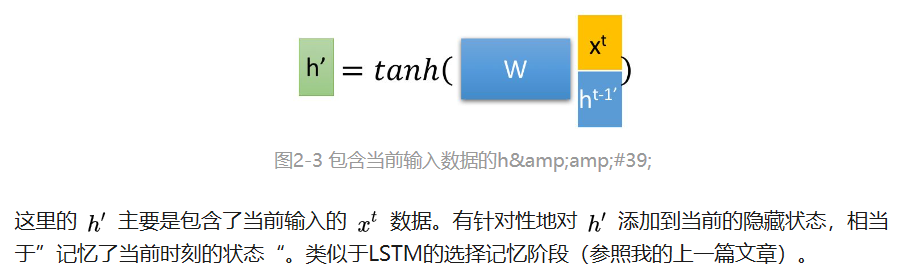然后就可以更新记忆了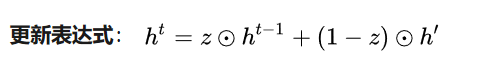首先再次强调一下，门控信号（这里的 z）的范围为0~1。门控信号越接近1，代表”记忆“下来的数据越多；而越接近0则代表”遗忘“的越多。

+前的式子表示表示对原本隐藏状态的选择性“遗忘”
+后的式子表示对包含当前节点信息的 h’进行选择性”记忆“

结合上述，这一步的操作就是忘记传递下来的ht-1中的某些维度信息，并加入当前节点输入的某些维度信息。
代码部分：
自己动手实现GRU的重点是初始化参数时，一共有11个参数需要生成：两个门控各三个，计算h’有三个，最后计算输出层还有两个。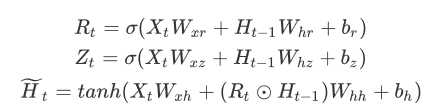初始化参数的代码：
num_inputs, num_hiddens, num_outputs = vocab_size, 256, vocab_size
print('will use', device)

def get_params():
def _one(shape):
ts = torch.tensor(np.random.normal(0, 0.01, size=shape), device=device, dtype=torch.float32) #正态分布
return torch.nn.Parameter(ts, requires_grad=True)
def _three():
return (_one((num_inputs, num_hiddens)),
_one((num_hiddens, num_hiddens)),
torch.nn.Parameter(torch.zeros(num_hiddens, device=device, dtype=torch.float32), requires_grad=True))

W_xz, W_hz, b_z = _three()  # 更新门参数
W_xr, W_hr, b_r = _three()  # 重置门参数
W_xh, W_hh, b_h = _three()  # 候选隐藏状态参数

# 输出层参数
W_hq = _one((num_hiddens, num_outputs))
b_q = torch.nn.Parameter(torch.zeros(num_outputs, device=device, dtype=torch.float32), requires_grad=True)
return nn.ParameterList([W_xz, W_hz, b_z, W_xr, W_hr, b_r, W_xh, W_hh, b_h, W_hq, b_q])

def init_gru_state(batch_size, num_hiddens, device):   #隐藏状态初始化
return (torch.zeros((batch_size, num_hiddens), device=device), )
初始化参数后就可以动手实现GRU了，就是将公式自行翻译一遍，然后训练模型
使用Pytorch简洁实现GRU就不用自己初始化模型和写GRU公式了，直接创建nn.GRU层
num_hiddens=256
num_epochs, num_steps, batch_size, lr, clipping_theta = 160, 35, 32, 1e2, 1e-2
pred_period, pred_len, prefixes = 40, 50, ['分开', '不分开']#前缀

lr = 1e-2 # 注意调整学习率
gru_layer = nn.GRU(input_size=vocab_size, hidden_size=num_hiddens)
model = d2l.RNNModel(gru_layer, vocab_size).to(device)
d2l.train_and_predict_rnn_pytorch(model, num_hiddens, vocab_size, device,
corpus_indices, idx_to_char, char_to_idx,
num_epochs, num_steps, lr, clipping_theta,
batch_size, pred_period, pred_len, prefixes)
长短期记忆LSTM
LSTM原理简介
和GRU一样，LSTM也是为了应对长序列中存在的梯度爆炸、梯度消失问题，同样也是运用了门控来控制每一个LSTM单元
其中重要的地方在于LSTM比起普通RNN多出一个状态C（记忆细胞）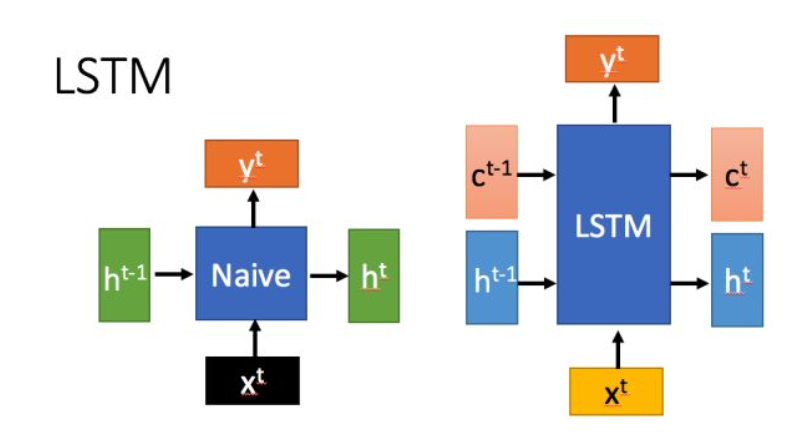接下来详细介绍LSTM的运作原理

首先使用LSTM的当前输入xt和上一个状态传递下来的ht-1拼接训练得到四个状态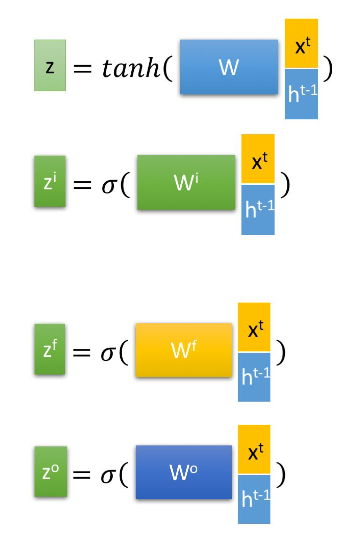其中z是作为状态ct的输入参数，下面三个分别是遗忘门、输入门、输出门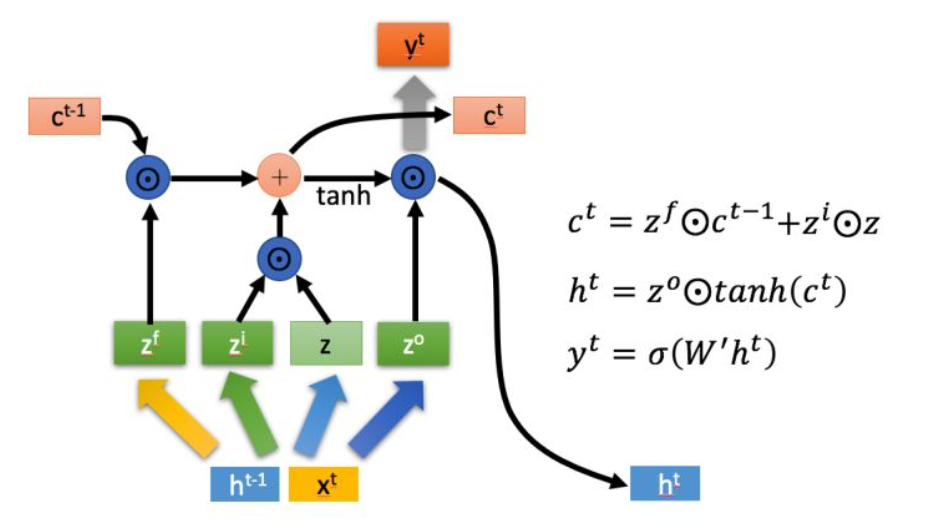通过遗忘门zf对上一时间的状态c进行选择性遗忘，然后拼接输入门zi和输入z计算当前的状态c，然后使用输出门配合tanh函数计算当前状态h

以上，就是LSTM的内部结构。通过门控状态来控制传输状态，记住需要长时间记忆的，忘记不重要的信息。总体来说就是三阶段：选择遗忘阶段、选择记忆阶段、输出阶段，而状态c是其中的中间状态
但由于训练开销的问题，很多时候会用效果差不多但是参数较少的GRU
代码方面：代码和GRU差不多，只是初始化参数时要初始化更多的参数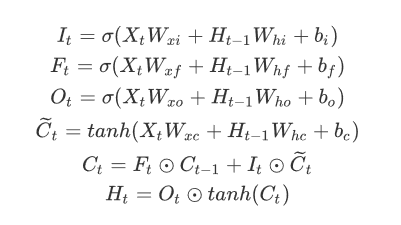这里的Ct上面以波浪线等同于上面的输入z
深度循环神经网络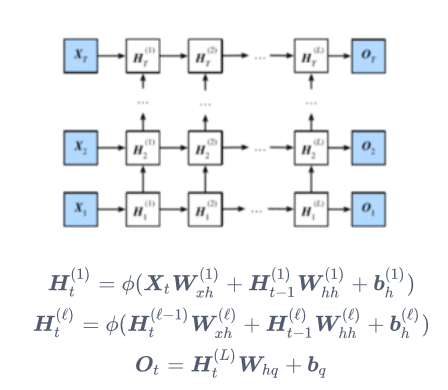相比于普通的RNN，深度循环神经网络相当于在中间多加了好几层，可以进行更为复杂的转换，代码只需要修改num_layersd的个数就行了
gru_layer = nn.LSTM(input_size=vocab_size, hidden_size=num_hiddens,num_layers=2)
但是层数越多并不一定效果会越好
双向循环神经网络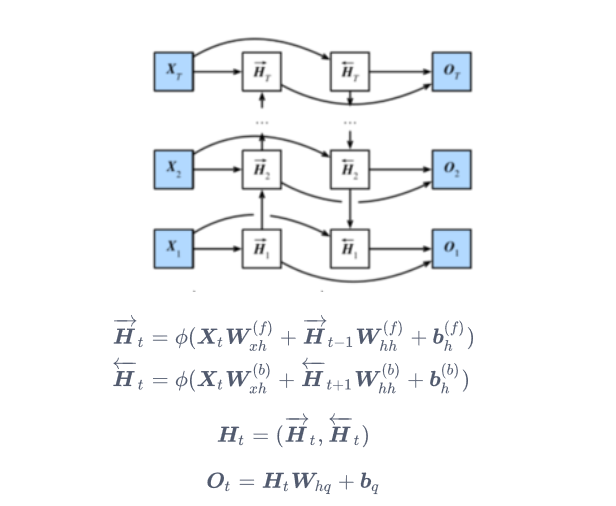除了存在从下往上的时序顺序之外，还存在着从上往下的时序顺序，最后把两个方向的Ht堆叠得到最终的Ht作为最后输出的输入，代码上只要指定bidirectional=True
gru_layer = nn.GRU(input_size=vocab_size, hidden_size=num_hiddens,bidirectional=True)


展开全文• 文章目录循环神经网络门控循环单元（GRU）门控循环单元重置门和更新门候选隐藏状态隐藏状态输出结果长短期记忆（LSTM）输入门、遗忘门和输出门候选记忆细胞记忆细胞隐藏状态输出结果深度循环神经网络双向循环神经...
文章目录循环神经网络门控循环单元（GRU）门控循环单元重置门和更新门候选隐藏状态隐藏状态输出结果长短期记忆（LSTM）输入门、遗忘门和输出门候选记忆细胞记忆细胞隐藏状态输出结果深度循环神经网络双向循环神经网络
循环神经网络
在循环神经网络中输入数据是存在时间相关性的，也就是说，前一个时间点的数据会对后一时间点的数据产生影响。假设 $\boldsymbol{X}_t \in \mathbb{R}^{n \times d}$ 是序列中时间步 $t$ 的小批量输入，$\boldsymbol{H}_t \in \mathbb{R}^{n \times h}$ 是该时间步的隐藏变量。与多层感知机不同的是，这里我们保存上一时间步的隐藏变量 $\boldsymbol{H}_{t-1}$，并引入一个新的权重参数 $\boldsymbol{W}_{hh} \in \mathbb{R}^{h \times h}$，该参数用来描述在当前时间步如何使用上一时间步的隐藏变量。具体来说，时间步 $t$ 的隐藏变量的计算由当前时间步的输入和上一时间步的隐藏变量共同决定：
$\boldsymbol{H}_t = \phi(\boldsymbol{X}_t \boldsymbol{W}_{xh} + \boldsymbol{H}_{t-1} \boldsymbol{W}_{hh} + \boldsymbol{b}_h).$
与多层感知机相比，我们在这里添加了 $\boldsymbol{H}_{t-1} \boldsymbol{W}_{hh}$ 一项。由上式中相邻时间步的隐藏变量 $\boldsymbol{H}_t$ 和 $\boldsymbol{H}_{t-1}$ 之间的关系可知，这里的隐藏变量能够捕捉截至当前时间步的序列的历史信息，就像是神经网络当前时间步的状态或记忆一样。因此，该隐藏变量也称为隐藏状态。隐藏状态中 $\boldsymbol{X}_t \boldsymbol{W}_{xh} + \boldsymbol{H}_{t-1} \boldsymbol{W}_{hh}$ 的计算等价于 $\boldsymbol{X}_t$与$\boldsymbol{H}_{t-1}$ 连结后的矩阵乘以$\boldsymbol{W}_{xh}$与$\boldsymbol{W}_{hh}$连结后的矩阵。由于隐藏状态在当前时间步的定义使用了上一时间步的隐藏状态，上式的计算是循环的。使用循环计算的网络即循环神经网络（recurrent neural network）。
循环神经网络有很多种不同的构造方法。含上式所定义的隐藏状态的循环神经网络是极为常见的一种。在时间步 $t$，输出层的输出和多层感知机中的计算类似：
$\boldsymbol{O}_t = \boldsymbol{H}_t \boldsymbol{W}_{hq} + \boldsymbol{b}_q.$
循环神经网络的参数包括隐藏层的权重 $\boldsymbol{W}_{xh} \in \mathbb{R}^{d \times h}$、$\boldsymbol{W}_{hh} \in \mathbb{R}^{h \times h}$ 和偏差 $\boldsymbol{b}_h \in \mathbb{R}^{1 \times h}$，以及输出层的权重 $\boldsymbol{W}_{hq} \in \mathbb{R}^{h \times q}$ 和偏差 $\boldsymbol{b}_q \in \mathbb{R}^{1 \times q}$。值得一提的是，即便在不同时间步，循环神经网络也始终使用这些模型参数。因此，循环神经网络模型参数的数量不随时间步的增加而增长。上图展示了循环神经网络在3个相邻时间步的计算逻辑。在时间步 $t$，隐藏状态的计算可以看成是将输入 $\boldsymbol{X}_t$ 和前一时间步隐藏状态 $\boldsymbol{H}_{t-1}$ 连结后输入一个激活函数为 $\phi$ 的全连接层。该全连接层的输出就是当前时间步的隐藏状态 $\boldsymbol{H}_t$，且模型参数为 $\boldsymbol{W}_{xh}$ 与 $\boldsymbol{W}_{hh}$ 的连结，偏差为 $\boldsymbol{b}_h$。当前时间步 $t$ 的隐藏状态 $\boldsymbol{H}_t$ 将参与下一个时间步 $t+1$ 的隐藏状态 $\boldsymbol{H}_{t+1}$ 的计算，并输入到当前时间步的全连接输出层。
门控循环单元（GRU）
循环神经网络中如何通过时间反向传播？一文中介绍了循环神经网络中的梯度计算方法。我们发现，当时间步数较大或者时间步较小时，循环神经网络的梯度较容易出现衰减或爆炸。虽然裁剪梯度可以应对梯度爆炸，但无法解决梯度衰减的问题。通常由于这个原因，循环神经网络在实际中较难捕捉时间序列中时间步距离较大的依赖关系。
门控循环单元
门控循环神经网络（gated recurrent neural network）的提出，正是为了更好地捕捉时间序列中时间步距离较大的依赖关系。它通过可以学习的门来控制信息的流动。其中，门控循环单元（gated recurrent unit，GRU）是一种常用的门控循环神经网络。
重置门和更新门
如下图所示，门控循环单元中的重置门和更新门的输入均为当前时间步输入 $\boldsymbol{X}_t$ 与上一时间步隐藏状态 $\boldsymbol{H}_{t-1}$，输出由激活函数为 sigmoid 函数的全连接层计算得到。具体来说，假设样本数为 $n$、输入个数为 $d$（即每个样本包含的元素数，一般为词典大小）、隐藏单元个数为 $h$，给定时间步 $t$ 的小批量输入 $\boldsymbol{X}_t \in \mathbb{R}^{n \times d}$ 和上一时间步隐藏状态 $\boldsymbol{H}_{t-1} \in \mathbb{R}^{n \times h}$。重置门 $\boldsymbol{R}_t \in \mathbb{R}^{n \times h}$ 和更新门 $\boldsymbol{Z}_t \in \mathbb{R}^{n \times h}$ 的计算如下：
$\boldsymbol{R}_t = \sigma(\boldsymbol{X}_t \boldsymbol{W}_{xr} + \boldsymbol{H}_{t-1} \boldsymbol{W}_{hr} + \boldsymbol{b}_r)$
$\boldsymbol{Z}_t = \sigma(\boldsymbol{X}_t \boldsymbol{W}_{xz} + \boldsymbol{H}_{t-1} \boldsymbol{W}_{hz} + \boldsymbol{b}_z)$
其中 $\boldsymbol{W}_{xr}, \boldsymbol{W}_{xz} \in \mathbb{R}^{d \times h}$ 和 $\boldsymbol{W}_{hr}, \boldsymbol{W}_{hz} \in \mathbb{R}^{h \times h}$ 是权重参数，$\boldsymbol{b}_r, \boldsymbol{b}_z \in \mathbb{R}^{1 \times h}$ 是偏差参数。sigmoid 函数可以将元素的值变换到0和1之间。因此，重置门 $\boldsymbol{R}_t$ 和更新门 $\boldsymbol{Z}_t$ 中每个元素的值域都是$[0, 1]$。
候选隐藏状态
接下来，门控循环单元将计算候选隐藏状态来辅助稍后的隐藏状态计算。如下图所示，我们将当前时间步重置门的输出与上一时间步隐藏状态做按元素乘法（符号为$\odot$）。如果重置门中元素值接近0，那么意味着重置对应隐藏状态元素为0，即丢弃上一时间步的隐藏状态。如果元素值接近1，那么表示保留上一时间步的隐藏状态。然后，将按元素乘法的结果与当前时间步的输入连结，再通过含激活函数 tanh 的全连接层计算出候选隐藏状态，其所有元素的值域为$[-1, 1]$。具体来说，时间步 $t$ 的候选隐藏状态 $\tilde{\boldsymbol{H}}_t \in \mathbb{R}^{n \times h}$ 的计算为：
$\tilde{\boldsymbol{H}}_t = \text{tanh}(\boldsymbol{X}_t \boldsymbol{W}_{xh} + \left(\boldsymbol{R}_t \odot \boldsymbol{H}_{t-1}\right) \boldsymbol{W}_{hh} + \boldsymbol{b}_h)$
其中 $\boldsymbol{W}_{xh} \in \mathbb{R}^{d \times h}$ 和 $\boldsymbol{W}_{hh} \in \mathbb{R}^{h \times h}$ 是权重参数，$\boldsymbol{b}_h \in \mathbb{R}^{1 \times h}$ 是偏差参数。从上面这个公式可以看出，重置门控制了上一时间步的隐藏状态如何流入当前时间步的候选隐藏状态。而上一时间步的隐藏状态可能包含了时间序列截至上一时间步的全部历史信息。因此，重置门可以用来丢弃与预测无关的历史信息从而有助于捕捉时间序列里短期的依赖关系
隐藏状态
最后，时间步 $t$ 的隐藏状态 $\boldsymbol{H}_t \in \mathbb{R}^{n \times h}$ 的计算使用当前时间步的更新门 $\boldsymbol{Z}_t$ 来对上一时间步的隐藏状态 $\boldsymbol{H}_{t-1}$ 和当前时间步的候选隐藏状态 $\tilde{\boldsymbol{H}}_t$ 做组合：
$\boldsymbol{H}_t = \boldsymbol{Z}_t \odot \boldsymbol{H}_{t-1} + (1 - \boldsymbol{Z}_t) \odot \tilde{\boldsymbol{H}}_t$值得注意的是，更新门可以控制隐藏状态应该如何被包含当前时间步信息的候选隐藏状态所更新，如上图所示。假设更新门在时间步 $t'$ 到 $t$（$t' < t$）之间一直近似于1。那么，在时间步 $t'$ 到 $t$ 之间的输入信息几乎没有流入时间步 $t$ 的隐藏状态 $\boldsymbol{H}_t$。实际上，这可以看作是较早时刻的隐藏状态 $\boldsymbol{H}_{t'-1}$ 一直通过时间保存并传递至当前时间步 $t$。这个设计可以应对循环神经网络中的梯度衰减问题，并更好地捕捉时间序列中时间步距离较大的依赖关系（即长期的依赖关系）。
输出结果
将上面得到的隐藏状态 $H_t$ 输入神经网络，得到结果 $\boldsymbol{Y}_t \in \mathbb{R}^{n \times q}$：
$\boldsymbol{Y}_t = \boldsymbol{H}_t \boldsymbol{W}_{hq} + \boldsymbol{b}_q$
总结来说：

重置门有助于捕捉时间序列里短期的依赖关系；
更新门有助于捕捉时间序列里长期的依赖关系。

长短期记忆（LSTM）
LSTM 中引入了3个门，即输入门（input gate）、遗忘门（forget gate）和输出门（output gate），以及与隐藏状态形状相同的记忆细胞（某些文献把记忆细胞当成一种特殊的隐藏状态），从而记录额外的信息。
输入门、遗忘门和输出门
与门控循环单元中的重置门和更新门一样，如下图所示，长短期记忆的门的输入均为当前时间步输入$\boldsymbol{X}_t$ 与上一时间步隐藏状态 $\boldsymbol{H}_{t-1}$，输出由激活函数为 sigmoid 函数的全连接层计算得到。如此一来，这3个门元素的值域均为$[0,1]$。具体来说，假设样本数为 $n$，输入个数为 $d$，隐藏单元个数为 $h$，给定时间步 $t$ 的小批量输入 $\boldsymbol{X}_t \in \mathbb{R}^{n \times d}$ 和上一时间步隐藏状态 $\boldsymbol{H}_{t-1} \in \mathbb{R}^{n \times h}$。 时间步 $t$ 的输入门 $\boldsymbol{I}_t \in \mathbb{R}^{n \times h}$、遗忘门 $\boldsymbol{F}_t \in \mathbb{R}^{n \times h}$ 和输出门 $\boldsymbol{O}_t \in \mathbb{R}^{n \times h}$ 分别计算如下：
$\boldsymbol{I}_t = \sigma(\boldsymbol{X}_t \boldsymbol{W}_{xi} + \boldsymbol{H}_{t-1} \boldsymbol{W}_{hi} + \boldsymbol{b}_i)$
$\boldsymbol{F}_t = \sigma(\boldsymbol{X}_t \boldsymbol{W}_{xf} + \boldsymbol{H}_{t-1} \boldsymbol{W}_{hf} + \boldsymbol{b}_f)$
$\boldsymbol{O}_t = \sigma(\boldsymbol{X}_t \boldsymbol{W}_{xo} + \boldsymbol{H}_{t-1} \boldsymbol{W}_{ho} + \boldsymbol{b}_o)$
其中的 $\boldsymbol{W}_{xi}, \boldsymbol{W}_{xf}, \boldsymbol{W}_{xo} \in \mathbb{R}^{d \times h}$ 和 $\boldsymbol{W}_{hi}, \boldsymbol{W}_{hf}, \boldsymbol{W}_{ho} \in \mathbb{R}^{h \times h}$ 是权重参数，$\boldsymbol{b}_i, \boldsymbol{b}_f, \boldsymbol{b}_o \in \mathbb{R}^{1 \times h}$ 是偏差参数。
候选记忆细胞
接下来，长短期记忆需要计算候选记忆细胞 $\tilde{\boldsymbol{C}}_t$。它的计算与上面介绍的3个门类似，但使用了值域在$[-1, 1]$的 tanh 函数作为激活函数，如下图所示。具体来说，时间步 $t$ 的候选记忆细胞 $\tilde{\boldsymbol{C}}_t \in \mathbb{R}^{n \times h}$ 的计算为
$\tilde{\boldsymbol{C}}_t = \text{tanh}(\boldsymbol{X}_t \boldsymbol{W}_{xc} + \boldsymbol{H}_{t-1} \boldsymbol{W}_{hc} + \boldsymbol{b}_c)$
其中 $\boldsymbol{W}_{xc} \in \mathbb{R}^{d \times h}$ 和 $\boldsymbol{W}_{hc} \in \mathbb{R}^{h \times h}$ 是权重参数，$\boldsymbol{b}_c \in \mathbb{R}^{1 \times h}$ 是偏差参数。
记忆细胞
我们可以通过元素值域在$[0, 1]$的输入门、遗忘门和输出门来控制隐藏状态中信息的流动，这一般也是通过使用按元素乘法（符号为$\odot$）来实现的。当前时间步记忆细胞 $\boldsymbol{C}_t \in \mathbb{R}^{n \times h}$ 的计算组合了上一时间步记忆细胞和当前时间步候选记忆细胞的信息，并通过遗忘门和输入门来控制信息的流动：
$\boldsymbol{C}_t = \boldsymbol{F}_t \odot \boldsymbol{C}_{t-1} + \boldsymbol{I}_t \odot \tilde{\boldsymbol{C}}_t.$
如下图所示，遗忘门控制上一时间步的记忆细胞 $\boldsymbol{C}_{t-1}$ 中的信息是否传递到当前时间步，而输入门则控制当前时间步的输入 $\boldsymbol{X}_t$ 通过候选记忆细胞 $\tilde{\boldsymbol{C}}_t$ 如何流入当前时间步的记忆细胞。如果遗忘门一直近似1且输入门一直近似0，过去的记忆细胞将一直通过时间保存并传递至当前时间步。这个设计可以应对循环神经网络中的梯度衰减问题，并更好地捕捉时间序列中时间步距离较大的依赖关系。隐藏状态
有了记忆细胞以后，接下来我们还可以通过输出门来控制从记忆细胞到隐藏状态 $\boldsymbol{H}_t \in \mathbb{R}^{n \times h}$ 的信息的流动：
$\boldsymbol{H}_t = \boldsymbol{O}_t \odot \text{tanh}(\boldsymbol{C}_t).$
这里的 tanh 函数确保隐藏状态元素值在-1到1之间。需要注意的是，当输出门近似1时，记忆细胞信息将传递到隐藏状态供输出层使用；当输出门近似0时，记忆细胞信息只自己保留。下图展示了长短期记忆中隐藏状态的计算。输出结果
长短期记忆的隐藏层输出包括隐藏状态和记忆细胞，但只有隐藏状态会传递到输出层，而记忆细胞不参与输出层的计算。
将上面得到的隐藏状态 $H_t$ 输入神经网络，得到结果 $\boldsymbol{Y}_t \in \mathbb{R}^{n \times q}$：
$\boldsymbol{Y}_t = \boldsymbol{H}_t \boldsymbol{W}_{hq} + \boldsymbol{b}_q$
深度循环神经网络
到目前为止所涉及的循环神经网络只有一个单向的隐藏层，在深度学习应用里，我们通常会用到含有多个隐藏层的循环神经网络，也称作深度循环神经网络。下图演示了一个有 $L$ 个隐藏层的深度循环神经网络，每个隐藏状态不断传递至当前层的下一时间步和当前时间步的下一层。在之前的介绍中，我们只包含输入层、第一层隐藏层和输出层。具体来说，在时间步 $t$ 里，设小批量输入 $\boldsymbol{X}_t \in \mathbb{R}^{n \times d}$（样本数为 $n$，输入个数为 $d$ ），第 $\ell$ 隐藏层（ $\ell=1,\ldots,L$ ）的隐藏状态为 $\boldsymbol{H}_t^{(\ell)} \in \mathbb{R}^{n \times h}$（隐藏单元个数为 $h$ ），输出层变量为 $\boldsymbol{O}_t \in \mathbb{R}^{n \times q}$（输出个数为 $q$ ），且隐藏层的激活函数为 $\phi$。第一层隐藏层的隐藏状态和之前的计算一样：
$\boldsymbol{H}_t^{(1)} = \phi(\boldsymbol{X}_t \boldsymbol{W}_{xh}^{(1)} + \boldsymbol{H}_{t-1}^{(1)} \boldsymbol{W}_{hh}^{(1)} + \boldsymbol{b}_h^{(1)}),$
其中权重 $\boldsymbol{W}_{xh}^{(1)} \in \mathbb{R}^{d \times h}$、$\boldsymbol{W}_{hh}^{(1)} \in \mathbb{R}^{h \times h}$ 和偏差  $\boldsymbol{b}_h^{(1)} \in \mathbb{R}^{1 \times h}$ 分别为第一层隐藏层的模型参数。
当 $1 < \ell \leq L$ 时，第 $\ell$ 隐藏层的隐藏状态的表达式为
$\boldsymbol{H}_t^{(\ell)} = \phi(\boldsymbol{H}_t^{(\ell-1)} \boldsymbol{W}_{xh}^{(\ell)} + \boldsymbol{H}_{t-1}^{(\ell)} \boldsymbol{W}_{hh}^{(\ell)} + \boldsymbol{b}_h^{(\ell)}),$
其中权重 $\boldsymbol{W}_{xh}^{(\ell)} \in \mathbb{R}^{h \times h}$、$\boldsymbol{W}_{hh}^{(\ell)} \in \mathbb{R}^{h \times h}$ 和偏差  $\boldsymbol{b}_h^{(\ell)} \in \mathbb{R}^{1 \times h}$ 分别为第 $\ell$ 隐藏层的模型参数。
最终，输出层的输出只需基于第 $L$ 隐藏层的隐藏状态：
$\boldsymbol{O}_t = \boldsymbol{H}_t^{(L)} \boldsymbol{W}_{hq} + \boldsymbol{b}_q,$
其中权重 $\boldsymbol{W}_{hq} \in \mathbb{R}^{h \times q}$ 和偏差 $\boldsymbol{b}_q \in \mathbb{R}^{1 \times q}$ 为输出层的模型参数。
同多层感知机一样，隐藏层个数 $L$ 和隐藏单元个数 $h$ 都是超参数。此外，如果将隐藏状态的计算换成门控循环单元或者长短期记忆的计算，我们可以得到深度门控循环神经网络。
双向循环神经网络
之前介绍的循环神经网络模型都是假设当前时间步是由前面的较早时间步的序列决定的，因此它们都将信息通过隐藏状态从前往后传递。有时候，当前时间步也可能由后面时间步决定。例如，当我们写下一个句子时，可能会根据句子后面的词来修改句子前面的用词。双向循环神经网络通过增加从后往前传递信息的隐藏层来更灵活地处理这类信息。下图演示了一个含单隐藏层的双向循环神经网络的架构。下面我们来介绍具体的定义。 给定时间步 $t$ 的小批量输入 $\boldsymbol{X}_t \in \mathbb{R}^{n \times d}$（样本数为 $n$，输入个数为 $d$ ）和隐藏层激活函数为 $\phi$。在双向循环神经网络的架构中， 设该时间步正向隐藏状态为$\overrightarrow{\boldsymbol{H}}_t \in \mathbb{R}^{n \times h}$（正向隐藏单元个数为 $h$ ）， 反向隐藏状态为 $\overleftarrow{\boldsymbol{H}}_t \in \mathbb{R}^{n \times h}$（反向隐藏单元个数为 $h$ ）。我们可以分别计算正向隐藏状态和反向隐藏状态：
\begin{aligned} \overrightarrow{\boldsymbol{H}}_t &= \phi(\boldsymbol{X}_t \boldsymbol{W}_{xh}^{(f)} + \overrightarrow{\boldsymbol{H}}_{t-1} \boldsymbol{W}_{hh}^{(f)} + \boldsymbol{b}_h^{(f)}),\ \overleftarrow{\boldsymbol{H}}_t &= \phi(\boldsymbol{X}_t \boldsymbol{W}_{xh}^{(b)} + \overleftarrow{\boldsymbol{H}}_{t+1} \boldsymbol{W}_{hh}^{(b)} + \boldsymbol{b}_h^{(b)}) \end{aligned}
其中权重 $\boldsymbol{W}_{xh}^{(f)} \in \mathbb{R}^{d \times h}$、$\boldsymbol{W}_{hh}^{(f)} \in \mathbb{R}^{h \times h}$、$\boldsymbol{W}_{xh}^{(b)} \in \mathbb{R}^{d \times h}$、$\boldsymbol{W}_{hh}^{(b)} \in \mathbb{R}^{h \times h}$ 和偏差 $\boldsymbol{b}_h^{(f)} \in \mathbb{R}^{1 \times h}$、$\boldsymbol{b}_h^{(b)} \in \mathbb{R}^{1 \times h}$ 均为模型参数。
然后我们连结两个方向的隐藏状态 $\overrightarrow{\boldsymbol{H}}_t$ 和 $\overleftarrow{\boldsymbol{H}}_t$ 来得到隐藏状态 $\boldsymbol{H}_t \in \mathbb{R}^{n \times 2h}$，并将其输入到输出层。输出层计算输出 $\boldsymbol{O}_t \in \mathbb{R}^{n \times q}$（输出个数为 $q$ ）：
$\boldsymbol{O}_t = \boldsymbol{H}_t \boldsymbol{W}_{hq} + \boldsymbol{b}_q$
其中权重 $\boldsymbol{W}_{hq} \in \mathbb{R}^{2h \times q}$ 和偏差 $\boldsymbol{b}_q \in \mathbb{R}^{1 \times q}$ 为输出层的模型参数。不同方向上的隐藏单元个数也可以不同。


展开全文神经网络 算法
• 8. 深度循环神经网络 本章目前为止介绍的循环神经网络，仅有一个单向的隐藏层。而在深度学习应用中，通常会涉及含有多个隐藏层的循环神经网络，也称作深度循环神经网络。 对于一个有LLL个隐藏层的深度循环神经网络，...
8. 深度循环神经网络
本章目前为止介绍的循环神经网络，仅有一个单向的隐藏层。而在深度学习应用中，通常会涉及含有多个隐藏层的循环神经网络，也称作深度循环神经网络。
对于一个有$L$个隐藏层的深度循环神经网络，每个隐藏状态不断传递至当前层的下一时间步和当前时间步的下一层，如下图所示：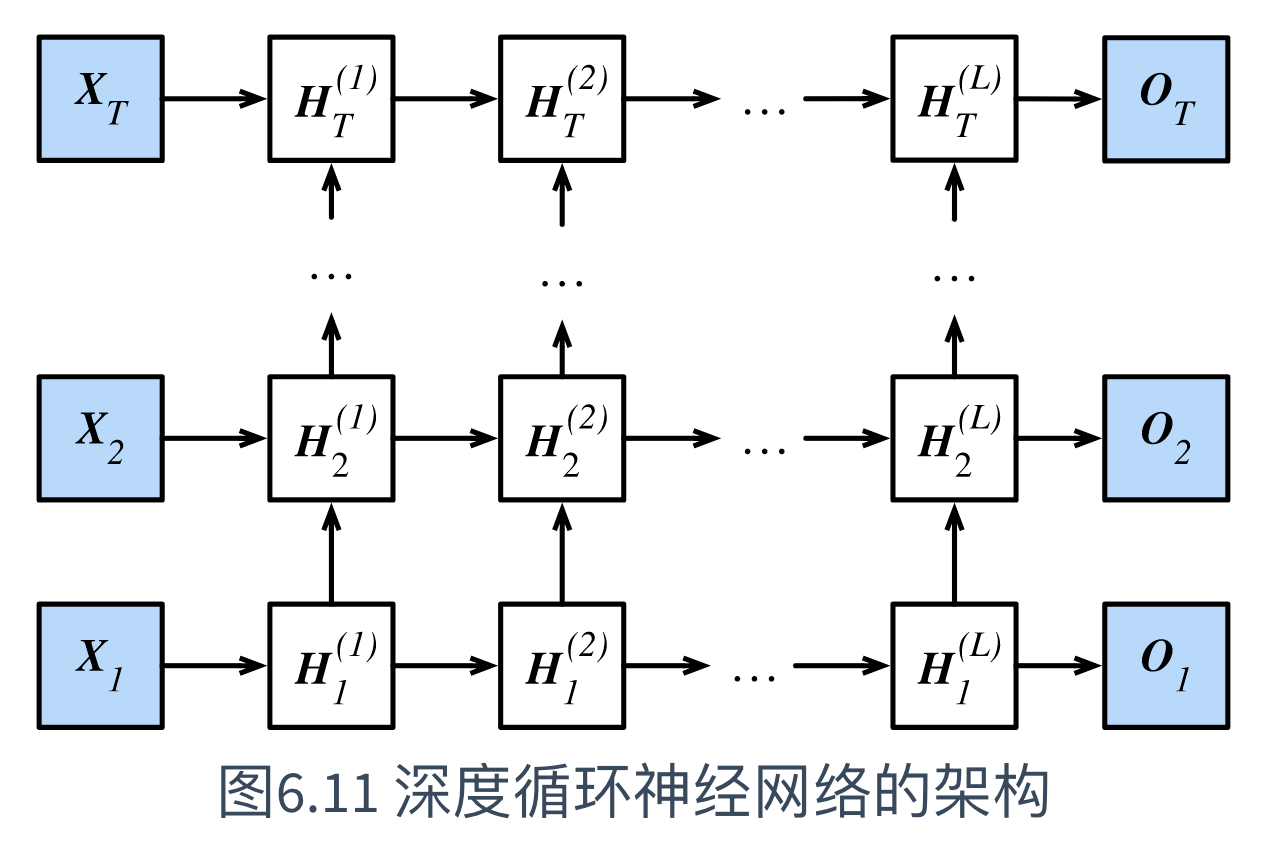具体来说，在时间步$t$里，设小批量输入$\boldsymbol{X}_t \in \mathbb{R}^{n \times d}$（样本数为$n$，输入个数为$d$），第$\ell$隐藏层（$\ell=1,\ldots,L$）的隐藏状态为$\boldsymbol{H}_t^{(\ell)} \in \mathbb{R}^{n \times h}$（隐藏单元个数为$h$），输出层变量为$\boldsymbol{O}_t \in \mathbb{R}^{n \times q}$（输出个数为$q$），且隐藏层的激活函数为$\phi$。
(1) 那么，有第1隐藏层的隐藏状态（同之前的计算）：
$\boldsymbol{H}_t^{(1)} = \phi(\boldsymbol{X}_t \boldsymbol{W}_{xh}^{(1)} + \boldsymbol{H}_{t-1}^{(1)} \boldsymbol{W}_{hh}^{(1)} + \boldsymbol{b}_h^{(1)})$
其中，权重$\boldsymbol{W}_{xh}^{(1)} \in \mathbb{R}^{d \times h}$、$\boldsymbol{W}_{hh}^{(1)} \in \mathbb{R}^{h \times h}$和偏差 $\boldsymbol{b}_h^{(1)} \in \mathbb{R}^{1 \times h}$分别为第1隐藏层的模型参数。
(2) 当$1 < \ell \leq L$时，有第$\ell$隐藏层的隐藏状态的表达式：
$\boldsymbol{H}_t^{(\ell)} = \phi(\boldsymbol{H}_t^{(\ell-1)} \boldsymbol{W}_{xh}^{(\ell)} + \boldsymbol{H}_{t-1}^{(\ell)} \boldsymbol{W}_{hh}^{(\ell)} + \boldsymbol{b}_h^{(\ell)})$
其中，权重$\boldsymbol{W}_{xh}^{(\ell)} \in \mathbb{R}^{h \times h}$、$\boldsymbol{W}_{hh}^{(\ell)} \in \mathbb{R}^{h \times h}$和偏差 $\boldsymbol{b}_h^{(\ell)} \in \mathbb{R}^{1 \times h}$分别为第$\ell$隐藏层的模型参数。
(3) 最终，输出层的输出只需基于第$L$隐藏层的隐藏状态：
$\boldsymbol{O}_t = \boldsymbol{H}_t^{(L)} \boldsymbol{W}_{hq} + \boldsymbol{b}_q$
其中，权重$\boldsymbol{W}_{hq} \in \mathbb{R}^{h \times q}$和偏差$\boldsymbol{b}_q \in \mathbb{R}^{1 \times q}$为输出层的模型参数。

类似于多层感知机，隐藏层个数$L$和隐藏单元个数$h$都为超参数。
此外，若将隐藏状态的计算换成门控循环单元或者长短期记忆的计算，可以得到深度门控循环神经网络。

参考
《动手学深度学习》(TF2.0版)


展开全文• 6.9 深度循环神经网络本章到目前为止介绍的循环神经网络只有一个单向的隐藏层，在深度学习应用里，我们通常会用到含有多个隐藏层的循环神经网络，也称作深度循环神经网络。图6.11演示了一个有LL LL个隐藏层的深度...
• 用Tensorflow可以很方便的实现深度循环神经网络。不过训练速度慢、效果差。可能是我没有研究明白深度循环神经网络的正确用法。 用LSTM和GRU已经足够了。深度循环神经网络的算法，还是有待提高的。 import tensorflow...tensorflow
• 深度循环神经网络 循环神经网络只有一个单向的隐藏层，在深度学习应用里，我们通常会用到含有多个隐藏层的循环神经网络，也称作深度循环神经网络。 下图演示了一个有LLL个隐藏层的深度循环神经网络，每个隐藏状态...python 算法
• MXNet深度循环神经网络----含有2个隐藏层的循环神经网络（程序） 《动手学深度学习》第六章 第9节的练习题，个人解答。 在深度学习应用里，我们通常会用到含有多个隐藏层的循环神经网络，也称作深度循环神经网络。下...神经网络 自然语言处理
• 循环神经网络 一个简单的循环神经网络，它由输入层、一个隐藏层和一个输出层组成：   如果把上面有W的那个带箭头的圈去掉，它就变成了最普通的全连接神经网络。 x是一个向量，它表示输入层的值（这里面没有画...
• 为此提出一种新的基于深度循环神经网络（DRNN）和区域自适应合成过采样算法（RA-SMOTE）的组合式入侵检测模型（DRRS）。首先，RA-SMOTE 对数据集中低频攻击样本进行自适应区域划分，实现差别样本增量，从数据层面...网络安全 入侵检测 过采样算法
• 深度学习-41: 深度循环神经网络(Recurrent NN, RNN) 深度学习原理与实践(开源图书)-总目录 在图像分类和目标识别领域，基于前馈神经网络的深度学习模型表现优异，但是在语音识别和自然语音处理领域深度学习...递归神经网络 时间序列数据
• 深度循环神经网络用于时间序列预测原文： Deep Recurrent Neural Networks for TimeSeries PredictionProblem文章简介内容和后面内容极大重合，有点不知所云 感觉前面废话有点多 文章内容很晦涩摘要增加隐藏层or......